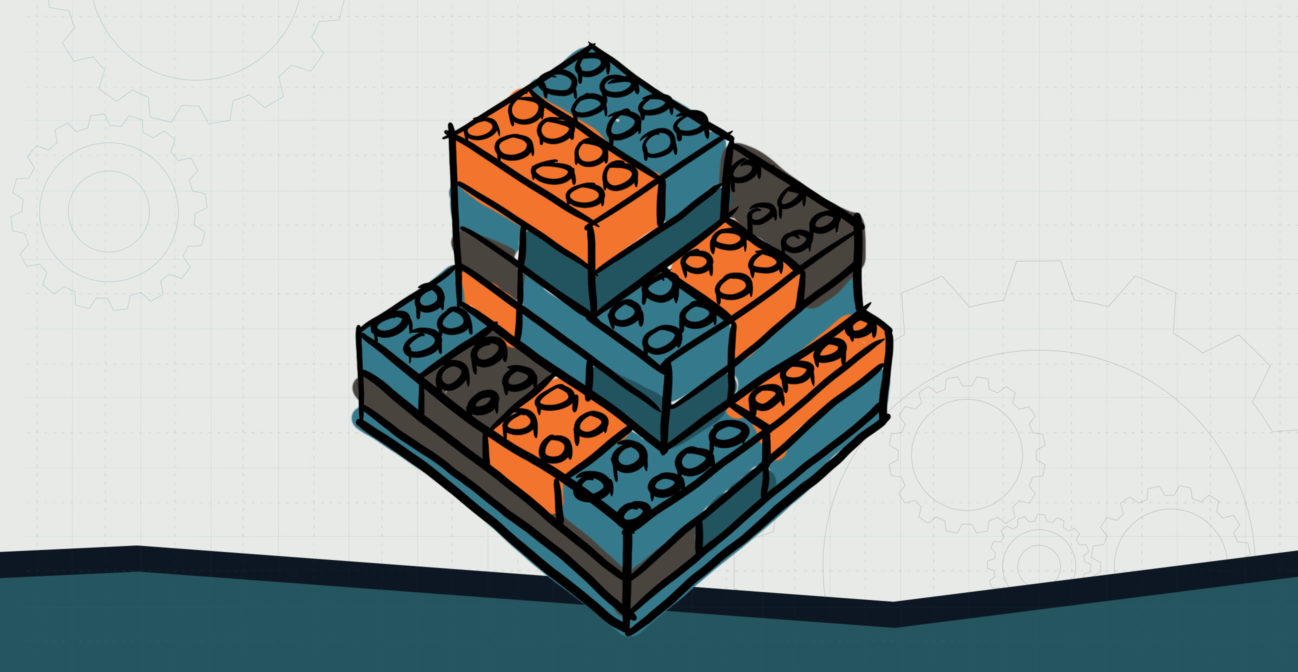# Pump Up the Volumes

## Design objects and investigate the volume of the objects.Objective:
Child will understand that volume is how you measure the amount of space something takes up.

Essential Question(s):
How do we measure how much space something takes up?

Special Materials:
Pencil and paper

Bricks Required:

16×16 or 8×8 plate, 2xN bricks

Project Structure

Engage/Explain:

1. Hold up a rectangular object of some sort (a ream of paper, a deck of cards, a book) and ask child how to measure it.
1. Child may recommend using a ruler. Ask what parts of the object should be measured. Child’s answers will vary.
2. Explain that child has already found the area of flat things, but that something like a book or deck of cards takes up space and that we measure that space through finding an object’s volume.
3. Measure the object to find its volume.

Explore:

1. Have child build a rectangular structure and find its volume.
1. Child could be restricted to just 2×2 and 2×4 bricks; total bricks might then be counted to find the volume (noting that 2×4 bricks would count as two bricks). Unit of measure would be “bricks.”
2. Child could build with any bricks and use the number of studs on each layer to find the volume. Unit of measure would be “cubic studs.”
3. The example has three sections: 8 on the top layer, 18 on the middle layer, and 32 on the bottom layer, yielding 58 cubic units.

Explain/Elaborate

1. Task child with finding the volume of quadrilateral containers (like a small cardboard box).
2. Have child devise an explanation on how they plan to measure the volume of the container.
1. Some possible methods are:
1. Using the SOHO bricks to fill the container;
2. Counting the brick units;
3. Using a ruler to measure the 3 dimensions.
3. Have child perform their method to determine the volume of the box.